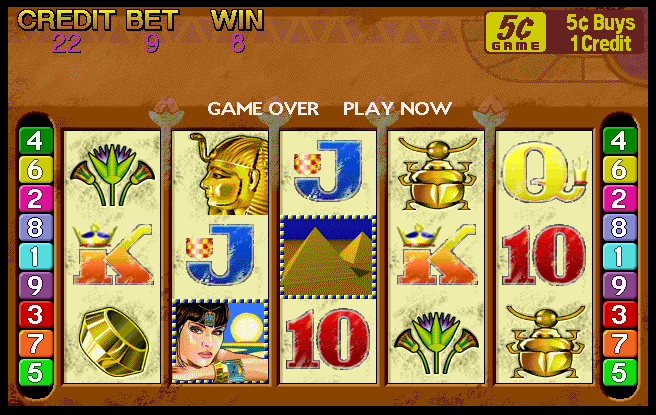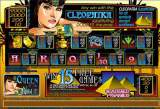# Queen of the Nile

## Slot Machine published 24 years ago by Aristocrat Leisure Industries Pty#### Listed and emulated in MAME.

Queen of the Nile © 1997 Aristocrat.

A 5-reel video slot machine with an Egyptian theme.Click to enlarge
(members only)
TECHNICAL/MACHINE PICT.
1

Aristocrat MK5 hardware. Also released on MK6 and MK7 hardware.

Game has 14 buttons.

Button panel, NSW 180 credit version :

Bet buttons : 1, 2, 5, 10, 20 credits per line
Line buttons : 1, 3, 5, 7, 9 lines
Extra buttons : Collect (yellow), Reserve (green), Gamble (purple) and Take Win/Start Feature (orange)

1, 2, 10 and 20 credit buttons are also used for Heart, Diamond, Club and Spade gamble respectively. This gamble is x4 if correct.
1 and 9 line buttons are used for red/black double up.

Button panel, NSW 500 credit version :

Bet buttons : 1, 5, 10, 20, 25 credits per line
Line buttons : 1, 5, 10, 15, 20 lines
Extra buttons : Collect (yellow), Reserve (green), Gamble (purple) and Take Win/Start Feature (orange)

1, 5, 20 and 25 credit buttons are also used for Heart, Diamond, Club and Spade gamble respectively. This gamble is x4 if correct.
1 and 20 line buttons are used for red/black double up.

Button panel, New Zealand 45 credit version :

Bet buttons : 1, 2, 3, 4, 5 credits per line
Line buttons : 1, 3, 5, 7/Autoplay, 9 lines
Extra buttons : Collect (yellow), Reserve (green), Gamble (purple) and Take Win/Start Feature (orange)

1, 2, 4 and 5 credit buttons are also used for Heart, Diamond, Club and Spade gamble respectively. This gamble is x4 if correct.
1 and 9 line buttons are used for red/black double up. An Autoplay button (green) replaces the Play 7 Lines button if Autoplay is enabled.

Button panel, Peru 90 credit version :

Bet buttons : 1, 2, 3, 5, 10 credits per line
Line buttons : 1, 3, 5, 7, 9 lines
Extra buttons : Collect (yellow), Reserve (green), Gamble (purple) and Take Win/Start Feature (orange)

1, 2, 5 and 10 credit buttons are also used for Heart, Diamond, Club and Spade gamble respectively. This gamble is x4 if correct.
1 and 9 line buttons are used for red/black double up.

Button panel, Victorian 100 credit version :

Bet buttons : 1, 2, 3, 4, 5 credits per line
Line buttons : 1, 5, 10, 15, 20 lines
Extra buttons : Collect (yellow), Reserve (green), Gamble (purple) and Take Win/Start Feature (orange)

1, 2, 4 and 5 credit buttons are also used for Heart, Diamond, Club and Spade gamble respectively. This gamble is x4 if correct.
1 and 20 line buttons are used for red/black double up.

Button panel, USA 90 credit version :

Line buttons : 1, 3, 5, 7, 9 lines (red)
Bet buttons : 1, 2, 3, 5, 10 credits per line
Extra buttons : Cashout (yellow), Service (red), Gamble (green) and Take Win (purple)

1 and 10 credit buttons are used for red/black double up (if applicable). Play 7 Lines is also used for the Start Feature button.

Button panel, USA 200 credit version :

Line buttons : 1, 5, 10, 15, 20 lines (red)
Bet buttons : 1, 2, 3, 5, 10 credits per line
Extra buttons : Cashout (yellow), Service (red), Gamble (green) and Take Win (purple)

1 and 10 credit buttons are used for red/black double up (if applicable). Play 15 Lines is also used for the Start Feature button.

TRIVIA

Queen of the Nile was released in May 1997.

Graphically identical to "Golden Pyramids", this game boasts "new" better sounding music which was used for every MKV game since.

This game is said to be the most popular game made by Aristocrat.

The MK5 casino versions have a graphical bug where the wrong reel background is used when a Beetle, Flower or Eye is flashing, which causes the background dust to be in the wrong position when the symbols flash.

SCORING

All pays are left to right except scattered Pyramids which pay any.

Cleopatra substitutes for all symbols except Pyramid and doubles the prize when substituting.

Paytable (standard version) :

Cleopatra : 2 = 10, 3 = 200, 4 = 2000, 5 = 9000
Statue : 2 = 2, 3 = 25, 4 = 100, 5 = 750
Bracelet : 2 = 2, 3 = 25, 4 = 100, 5 = 750
Beetle : 3 = 15, 4 = 100, 5 = 400
Eye : 3 = 10, 4 = 75, 5 = 250
Flower: 3 = 10, 4 = 50, 5 = 250
Ace : 3 = 10, 4 = 50, 5 = 125
King : 3 = 5, 4 = 50, 5 = 100
Queen : 3 = 5, 4 = 25, 5 = 100
Jack : 3 = 5, 4 = 25, 5 = 100
Ten : 3 = 5, 4 = 25, 5 = 100
Nine : 2 = 2, 3 = 5, 4 = 25, 5 = 100
Pyramid : 2 = 2, 3 = 5, 4 = 20, 5 = 400

Paytable (French version, bet 1-8 credits) :

Cleopatra : 2 = 10, 3 = 200, 4 = 2000, 5 = 9000
Statue : 2 = 2, 3 = 25, 4 = 100, 5 = 750
Bracelet : 2 = 2, 3 = 25, 4 = 100, 5 = 750
Beetle : 3 = 15, 4 = 100, 5 = 400
Eye : 3 = 10, 4 = 75, 5 = 250
Flower: 3 = 10, 4 = 50, 5 = 250
Ace : 3 = 10, 4 = 50, 5 = 125
King : 3 = 5, 4 = 50, 5 = 100
Queen : 3 = 5, 4 = 25, 5 = 100
Jack : 3 = 5, 4 = 25, 5 = 100
Ten : 3 = 5, 4 = 25, 5 = 100
Nine : 2 = 2, 3 = 5, 4 = 25, 5 = 100
Pyramid : 2 = 2, 3 = 5, 4 = 20, 5 = 400

Paytable (French version, bet 9-27 credits) :

Cleopatra : 2 = 10, 3 = 200, 4 = 2000, 5 = 10000
Statue : 2 = 2, 3 = 25, 4 = 100, 5 = 800
Bracelet : 2 = 2, 3 = 25, 4 = 100, 5 = 800
Beetle : 3 = 15, 4 = 100, 5 = 500
Eye : 3 = 10, 4 = 75, 5 = 350
Flower: 3 = 10, 4 = 50, 5 = 350
Ace : 3 = 10, 4 = 50, 5 = 175
King : 3 = 5, 4 = 50, 5 = 125
Queen : 3 = 5, 4 = 25, 5 = 125
Jack : 3 = 5, 4 = 25, 5 = 125
Ten : 3 = 5, 4 = 25, 5 = 125
Nine : 2 = 2, 3 = 5, 4 = 25, 5 = 125
Pyramid : 2 = 2, 3 = 5, 4 = 20, 5 = 400

Paytable (New Zealand version) :

Cleopatra : 2 = 10, 3 = 200, 4 = 500, 5 = 1000
Statue : 2 = 2, 3 = 25, 4 = 100, 5 = 250
Bracelet : 2 = 2, 3 = 25, 4 = 100, 5 = 250
Beetle : 3 = 15, 4 = 100, 5 = 200
Eye : 3 = 10, 4 = 75, 5 = 150
Flower: 3 = 10, 4 = 50, 5 = 150
Ace : 3 = 10, 4 = 50, 5 = 125
King : 3 = 5, 4 = 50, 5 = 100
Queen : 3 = 5, 4 = 25, 5 = 100
Jack : 3 = 5, 4 = 25, 5 = 100
Ten : 3 = 5, 4 = 25, 5 = 100
Nine : 2 = 2, 3 = 5, 4 = 25, 5 = 100
Pyramid : 2 = 2, 3 = 5, 4 = 20, 5 = 200

Paytable (USA version) :

Cleopatra : 2 = 10, 3 = 150, 4 = 1500, 5 = 3000
Statue : 2 = 2, 3 = 25, 4 = 150, 5 = 1500
Bracelet : 2 = 2, 3 = 15, 4 = 100, 5 = 750
Beetle : 3 = 15, 4 = 75, 5 = 375
Eye : 3 = 10, 4 = 75, 5 = 250
Flower: 3 = 10, 4 = 50, 5 = 200
Ace : 3 = 10, 4 = 50, 5 = 125
King : 3 = 5, 4 = 40, 5 = 100
Queen : 3 = 5, 4 = 25, 5 = 100
Jack : 3 = 5, 4 = 25, 5 = 100
Ten : 3 = 5, 4 = 25, 5 = 100
Nine : 2 = 2, 3 = 5, 4 = 25, 5 = 100
Pyramid : 2 = 2, 3 = 5, 4 = 20, 5 = 400

TIPS AND TRICKS

WIN 15 FREE GAMES : 15 free games are won with 3x, 4x or 5x scattered Pyramids. All prizes are tripled during the free games. Free games can be won again during the feature. Bets stakes and lines played are the same as the game that started the feature.

SERIES

1) Dolphin Treasure (1996)
2) Beyond the Reef (1997)
3) Golden Pyramids (1997)
4) Queen of the Nile (1997)
6) Flame of Olympus (1998)
7) Geisha (2000)
8) Torch of the Gods (2001)
9) Queen of the Nile Special Edition (2002)
10) Golden Pyramids Special Edition (2002)
11) Geisha [Power Pay] (2008)
12) Love on the Nile (2011)
13) Dolphin Treasure Legends (2012)
14) Queen of the Nile Legends (2013)
15) Geisha Legends (2013)

PORTS

OTHERS:
Online slot (2012) Queen of the Nile

SOURCES

Slot's ROM.
Slot's picture.